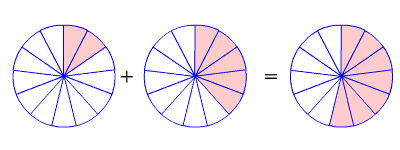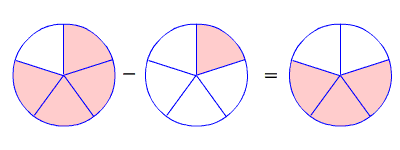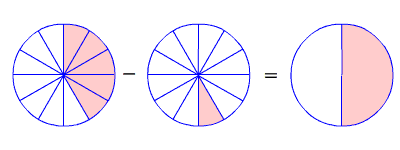# Adding and Subtracting Fractions with Like Denominators

When adding and subtracting fractions, the first thing to check is if the denominators are the same.

If the denominators are the same, then it's pretty easy: just add or subtract the numerators , and write the result over the same denominator.

$\frac{2}{13}+\frac{5}{13}=\frac{7}{13}$$\frac{4}{5}-\frac{1}{5}=\frac{3}{5}$You may get an answer which is not in lowest terms , even if the fractions you were adding and subtracting both were. In this case, you have to reduce the fraction .

$\frac{5}{12}+\frac{1}{12}=\frac{6}{12}=\frac{6\text{\hspace{0.17em}}÷\text{\hspace{0.17em}}6}{12\text{\hspace{0.17em}}÷\text{\hspace{0.17em}}6}=\frac{1}{2}$